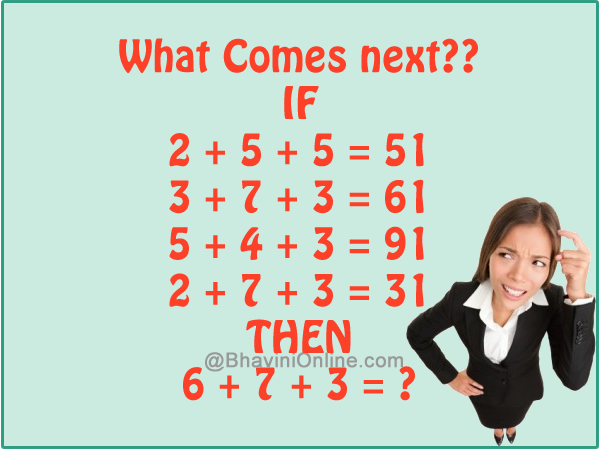# What Comes Next in the Sequence: IF 2 + 5 + 5 = 51, Then 6 + 7 + 3 = ?

What Comes next in the sequence;

IF
2 + 5 + 5 = 51
3 + 7 + 3 = 61
5 + 4 + 3 = 91
2 + 7 + 3 = 31
THEN
6 + 7 + 3 = ?So were you able to solve the riddle? Leave your answers in the comment section below.